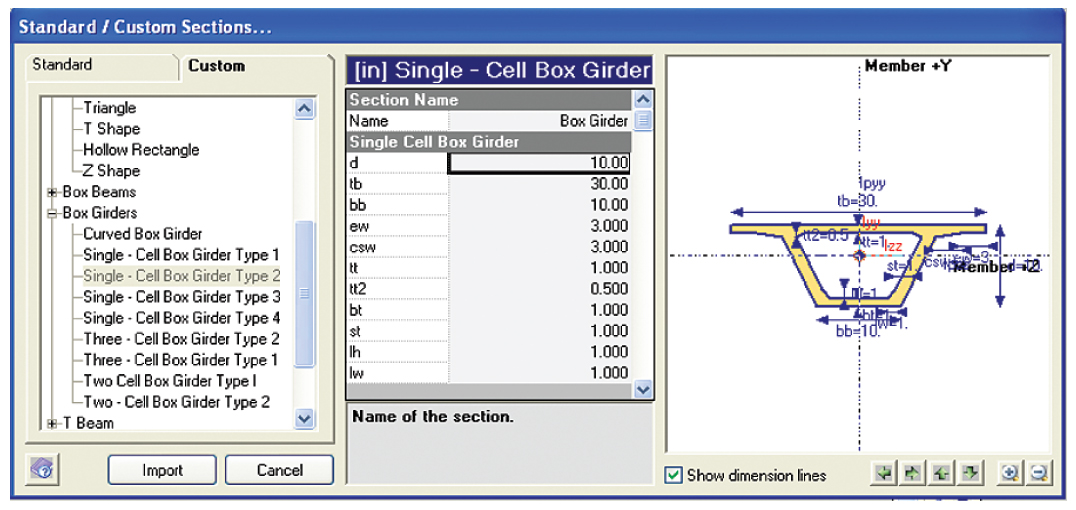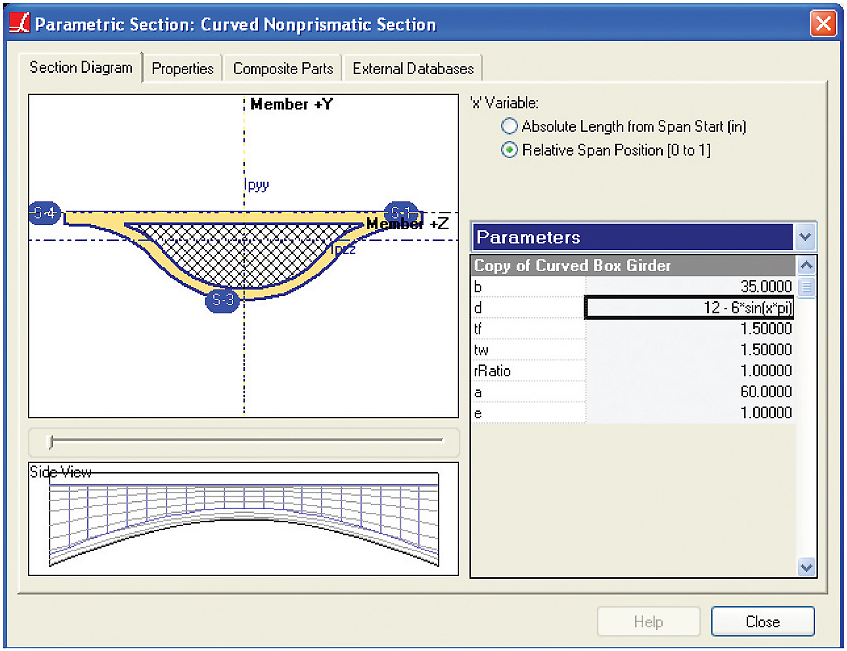## LARSA 4D Section Composer Tool

LARSA Section Composer is a graphical companion tool for modeling arbitrary sections for use in LARSA 4D. Section Composer supports standard, parametric, nonprismatic, and custom shape sections. It is able to compute section properties in real time.

## Parametric Definition

Sections in Section Composer are defined parametrically, meaning points are normally entered as equations of a few parameters, such width (b), depth (d), and thickness (t). Sections defined this way can be reused and resized as needed without re-computing the coordinates of control points. By simply changing a parameter, coordinates are immediately updated.

Each point on a shape's perimeter is defined using mathematical equations. Equations in terms of section parameters, such as for creating the points on a rectangle (d,b), (-d,b), (-d,-b), (d,-b), make it possible to alter section dimensions without modifying each point, and to apply nonprismatic variation according to any user-enterable formula, such as d + x/100 for a variation that starts at d and increases on a linear 1:100 slope.## Automatic Computation of Properties

Section Composer can be used to model cross-sections with holes, composite parts, and built-up parts with any arbitrary shape. Properties including area, moment of inertia, radius of gyration, and torsion constant are all computed by Section Composer for any shape.

## Nonpristmatic Variation

Accurate modeling of bridges requires the use of nonprismatic sections, sections whose dimensions vary along the length of the member. LARSA Section Composer makes it easy to define nonprismatic variation in sections by applying a formula to a parametric section definition. Formulas give the value of a parameter as a function of the position along the length of a span. Linear, parabolic, sinusoidal, and other types of functions can be attached to parameters, like depth, to control nonprismatic variation.